×

Html & Html5

Introduction to Html

Component of Html

Html Form

Html5 basics

Html5 Grid

Html5 Graphics

Html5 Media

Html5 APIs

Python and Pandas

Introduction to Python

Component of Python

Python Tuple

Tuple is another collection data type in Python . It is the collection which is ordered and unchangeable. In Python tuples are written with round brackets().
Examples:-

Create a tuple

thistuple = ("Banana", "Apple", "Mango")

print(thistuple)

Output of this Program

So here in above program we had given the command of creating Tuple data to our Python interpreter. Let’s see .So our ‘Tuple’ is been ready.

Access Items in Tuple

Here in this data type also , if we want to access any particular elements from the tuple , than we can write the index number of specified element inside the square bracket.

Let’s understand this by an example :-

thislist = ('Banana', 'Apple', 'Mango')

print(thistuple)

Output of this Program

So here in the above program we just want to access only one item from the tuple who is having index no. 1, means ‘Apple’ we want because first one is included ‘0’ in Python. So let’s see what output it gives :-
So let’s see what output it gives :-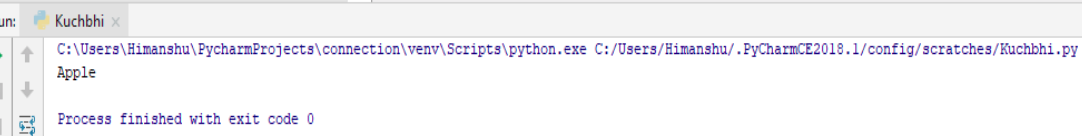Here it just show us the desired element which we want.

Change Tuple values

Once you creates a tuple ,than you cannot changes it’s values. Tuple are unchangeable:

Let’s understand this by an example :-

thistuple = ('Banana', 'Apple' , 'Mango')

thistuple = 'Litchi'

print(thistuple)

Output of this Program

So here in the above Program we just gave command to our Python interpreter that we want to change the item , of index number ‘1’ from ‘Apple’ to ‘Litchi’ .
So let’s see what output it gives :-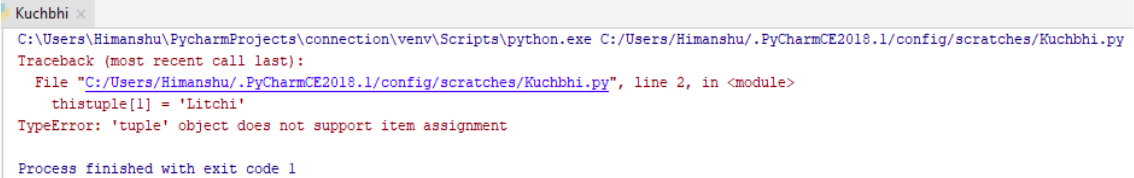It gives us the error because tuple is unchangeable collection data type that means we can’t change the values here.

Loops through Tuple

As we have earlier discussed too that loops are the repetition of the same ‘string’ , ‘item’ or etc. under the same condition . In Python we can loop through the tuple by using the ‘for’ loop .

Let’s understand this by an example :-

thistuple =('Banana', 'Apple' , 'Mango')

for x in thistuple:

print(X)

Output of this Program

So here in the above program we just gave command to our Python interpreter that we want to print all the items , one by one.
So let’s see what output it gives :-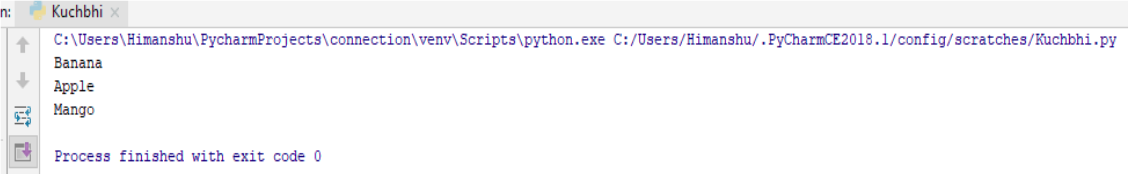Here it just shows all the elements one by one .

( More about Loops we will talk further on the looping section. )

Check if item or element exist in Tuple

We can also check items or elements in our tuple by using the ‘in’ keyword.
Let us understand this by the following example :-

thistuple = ('Banana', 'Apple', 'Mango')

if 'Mango' in thistuple :

print("Yes it is in thistuple")

Output of this ProgramSo our ‘Mango’ is in the tuple . So it just give the correct output.

( We will read in detail regarding ‘if’ statement . )

Methods in Tuple

As we know that methods are basically the function which we want to perform in our coding . In Python there are some inbuilt methods are there for Tuple too .
So let’s see some of them :-

The len() method - It just returns the length of the Tuple , base on their index number

thistuple = (‘Banana’, 'Apple', 'Mango')

print(len(thistuple))

Output of this ProgramSo here we just get the length of the tuple based on their index number.

Adding items on the Tuple - once you are created tuple ,you cannot access any item,Tuple is unchangeable.

thistuple=('Banana', 'Apple', 'Mango')

thistuple='Papaya' # This will raise an error

print(thistuple)

Output of this Program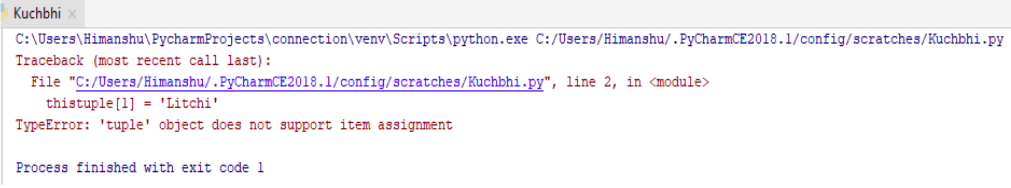Here in Tuple we can’t change or add any item or element.

Removing items in tuples you cannot remove the item in tuple, because tuple unchangeable but you can delete tuple is completely

thistuple=('Banana', 'Apple', 'Mango')

del(thistuple)

print(thistuple) #this will raise an error because the tuple no longer exists

Output of this Program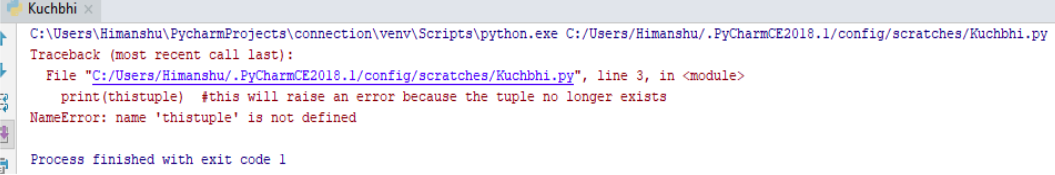So here through clear() method all the items or elements are gone.

The tuple constructor , tuple() can used to make a tuple

thistuple = tuple(('Apple', 'Banana', 'Mango'))

print(thistuple)

Output of this ProgramHere through tuple() method we have construct the tuple.

Some methods of Tuple and their functionality

There are only two method that tuple objects can calls: count and index. Also the page include built-in-functions can take tuple as a parameter and perform some task.
Here we are enlisting some methods of tuple and their description which we can use in Python.

Method Description
count() Returns occurrences of element in a tuple
index() Return smallest index of element in tuple.
any() Check if any iterable element is true
all() Returns true when lterable all element true
ascii() Returns String Containing Printable Representation
bool() Convert a value of Boolean
enumerate() Return enumerate object
filter() Construct iterable from element which are true
iter() Return iterable for an object
len() Return length for an object
max() Return largest element
min() Return smallest element
map() Applies function and return a list
reversed() Returns reversed iterator of a sequence.
slice() Create a slice object specified by range
sorted() Return sorted list from a given iterable.